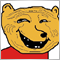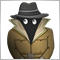# Calculate the distance between two moving averages48

Hi, I was in a form and still did not get the correct answer after the overall search.
I'm looking for a way to get the distance between the two pipelines between the two moving averages of 15 and 60
Is there anyone who can help me on this topic?
Thanks

```//+------------------------------------------------------------------+
//|                        Copyright 2017, MetaQuotes Software Corp. |
//|                                             https://www.mql5.com |
//+------------------------------------------------------------------+
#property version   "1.00"
#property strict
extern int MA1 = 15;
extern int MA2 = 60;
extern string Comment1 = "// 0 - PRICE_CLOSE, 1 - PRICE_OPEN, 2 - PRICE_HIGH, 3 - PRICE_LOW, 4 - PRICE_MEDIAN, 5 - PRICE_TYPICAL, 6 - PRICE_WEIGHTED";
extern int PriceType = 0;
extern string Comment2 = "// 0 - MODE_SMA, 1 - MODE_EMA, 2 - MODE_SMMA, 3 - MODE_LWMA";
extern int MA_MODE = 0;
extern int Tolerance = 5;
extern int Distance = 30;
extern double sVolume = 0.1;
double MA11=iMA(NULL,0,MA1,0,MA_MODE,PriceType,0);
double MA22=iMA(NULL,0,MA2,0,MA_MODE,PriceType,0);
int pipsMAsell = (int)MathFloor( MathAbs(MA22-MA11)/(_Point) );
int pipsMAbuy = (int)MathFloor( MathAbs(MA11-MA22)/(_Point) );
//+------------------------------------------------------------------+
//| Expert tick function                                             |
//+------------------------------------------------------------------+
void OnTick()
{
//---

{

}
else if(pipsMAsell == Tradepip && Close<MA11 && MA22<MA11)
{

Print("sell");

}

}
//+------------------------------------------------------------------+
```20207

aliebnehosseini: I'm looking for a way to get the distance between the two pipelines between the two moving averages of 15 and 60 Is there anyone who can help me on this topic?
2. `int pipsMAsell = (int)MathFloor( MathAbs(MA22-MA11)/(_Point) );`
A point is not a pip.
What is a TICK? - MQL4 and MetaTrader 4 - MQL4 programming forum

3. It is unlikely that the differences will be exactly your Tradepip. What if they are larger?48

whroeder1:
2. A point is not a pip.
What is a TICK? - MQL4 and MetaTrader 4 - MQL4 programming forum

3. It is unlikely that the differences will be exactly your Tradepip. What if they are larger?

hi whroeder1

Yes I found the code but it does not work.
My intention is, when the distance between the moving average of 15 and 60 is 20 pips, expert will begin to buy or sell, look carefully at the picture.

sorry i cannot speak english very well.1079

The wheel you're trying to reinvent is called MACD or Awesome Oscillator.7853

```//+------------------------------------------------------------------+
//|                        Copyright 2017, MetaQuotes Software Corp. |
//|                                             https://www.mql5.com |
//+------------------------------------------------------------------+
#property version   "1.00"
#property strict
extern int MA1 = 15;
extern int MA2 = 60;
extern string Comment1 = "// 0 - PRICE_CLOSE, 1 - PRICE_OPEN, 2 - PRICE_HIGH, 3 - PRICE_LOW, 4 - PRICE_MEDIAN, 5 - PRICE_TYPICAL, 6 - PRICE_WEIGHTED";
extern int PriceType = 0;
extern string Comment2 = "// 0 - MODE_SMA, 1 - MODE_EMA, 2 - MODE_SMMA, 3 - MODE_LWMA";
extern int MA_MODE = 0;
extern int Tolerance = 5;
extern int Distance = 30;
extern double sVolume = 0.1;
double MA11, MA22;
//+------------------------------------------------------------------+
//| Expert tick function                                             |
//+------------------------------------------------------------------+
void OnTick()
{
//---
MA11=iMA(NULL,0,MA1,0,MA_MODE,PriceType,0);
MA22=iMA(NULL,0,MA2,0,MA_MODE,PriceType,0);
pipsMAsell = (int)MathFloor( MathAbs(MA22-MA11)/(_Point) );

{